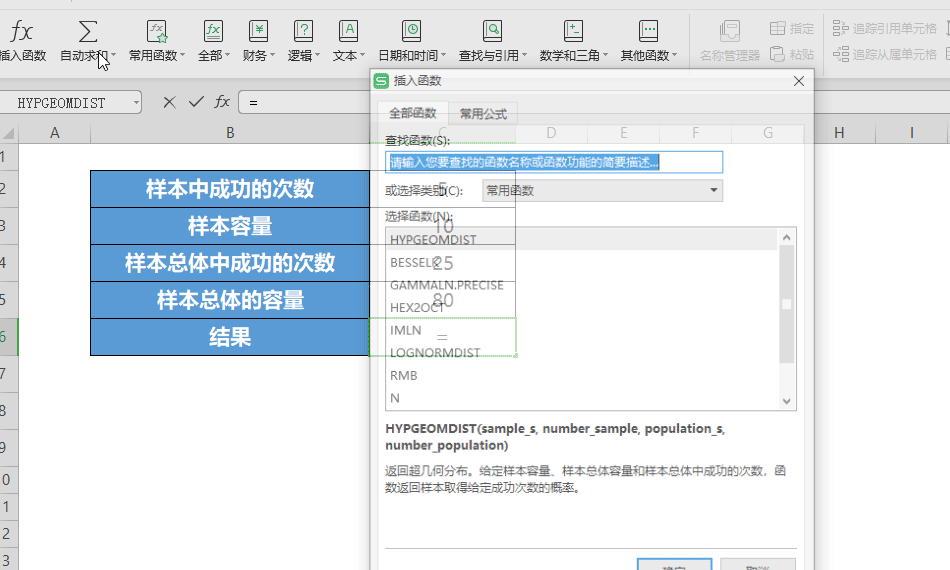## HYPGEOMDIST函数给定样本的超几何分布概率

HYPGEOMDIST(sample_s,number_sample,population_s,number_population)
Sample_s 样本中成功的次数。
Number_sample 样本容量。
Population_s 样本总体中成功的次数。
Number_population 样本总体的容量。

1、sample_s < 0 或 sample_s 大于 number_sample 和 population_s 中的较小值
2、sample_s 小于 0 或 (number_sample - number_population + population_s) 中的较大值
3、number_sample ≤ 0 或 number_sample > number_population
4、population_s ≤ 0 或 population_s > number_population
5、number_population ≤ 0x = sample_s
n = number_sample
M = population_s
N = number_population

HYPGEOMDIST函数是一个统计函数。￭首先打开表格，将光标定位在C6处，点击插入HYPGEOMDIST函数。